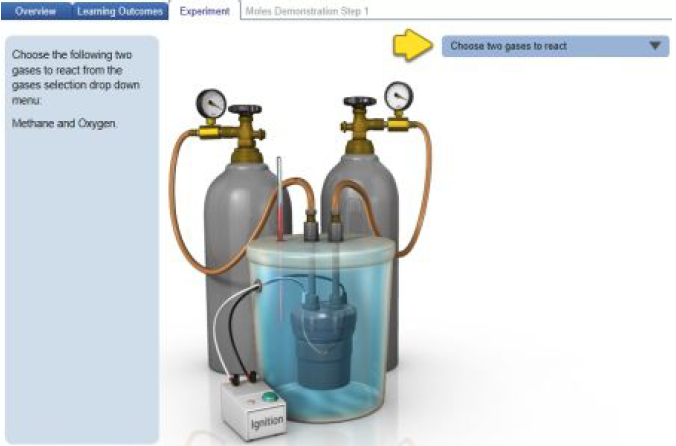# Problem: Assuming the temperature and volume remain constant, changes to the pressure in the reaction vessel will directly correspond to changes in the number of moles based on the ideal gas law (PV = nRT).Suppose the reaction between nitrogen and hydrogen was run according to the amounts presented in Part A (0.151 mol of N2 and 0.453 mol of H2), and the temperature and volume were constant at values of 303 K and 2.00 L, respectively. If the pressure was 7.51 atm prior to the reaction, what would be the expected pressure after the reaction was completed?Express the pressure in atmospheres to three significant digits.Balanced chemical equations include coefficients that describe the ratio between molecules or moles of reactants and products. This relationship would allow you to utilize pressure measurements to monitor the progress of a reaction between gases if the number of gas molecules on each side of the reaction is different. An example of a reaction that cannot be monitored in real time is the combustion of methane:CH4(g) + 2 O2(g) → CO2(g) + 2 H2O(g) + heatThe temperature at which the reaction takes place would result in the formation of water in the gas phase, which means that three moles of reactants (one mole of methane and two moles of oxygen) would form three moles of product (one mole of carbon dioxide and two moles of water vapor). However, the percent yield of a reaction could eventually be determined once the product mixture is permitted to cool, and the pressure will reflect the change in the number of moles of gas since water vapor will condense into the liquid phase and carbon dioxide would be the only product gas. We will explore several gas reactions in which the number of gaseous molecules differs between the reactants and products.Click on the image below to explore this simulation, which allows you run reactions between various gases and specify their amounts. When you click the simulation link, you will be able to select among Overview, Learning Outcomes, and Experiment tabs.

###### FREE Expert Solution
79% (453 ratings)View Complete Written Solution
###### Problem Details

Assuming the temperature and volume remain constant, changes to the pressure in the reaction vessel will directly correspond to changes in the number of moles based on the ideal gas law (PV = nRT).

Suppose the reaction between nitrogen and hydrogen was run according to the amounts presented in Part A (0.151 mol of N2 and 0.453 mol of H2), and the temperature and volume were constant at values of 303 K and 2.00 L, respectively. If the pressure was 7.51 atm prior to the reaction, what would be the expected pressure after the reaction was completed?

Express the pressure in atmospheres to three significant digits.

Balanced chemical equations include coefficients that describe the ratio between molecules or moles of reactants and products. This relationship would allow you to utilize pressure measurements to monitor the progress of a reaction between gases if the number of gas molecules on each side of the reaction is different. An example of a reaction that cannot be monitored in real time is the combustion of methane:

CH4(g) + 2 O2(g) → CO2(g) + 2 H2O(g) + heat

The temperature at which the reaction takes place would result in the formation of water in the gas phase, which means that three moles of reactants (one mole of methane and two moles of oxygen) would form three moles of product (one mole of carbon dioxide and two moles of water vapor). However, the percent yield of a reaction could eventually be determined once the product mixture is permitted to cool, and the pressure will reflect the change in the number of moles of gas since water vapor will condense into the liquid phase and carbon dioxide would be the only product gas. We will explore several gas reactions in which the number of gaseous molecules differs between the reactants and products.

Click on the image below to explore this simulation, which allows you run reactions between various gases and specify their amounts. When you click the simulation link, you will be able to select among Overview, Learning Outcomes, and Experiment tabs.